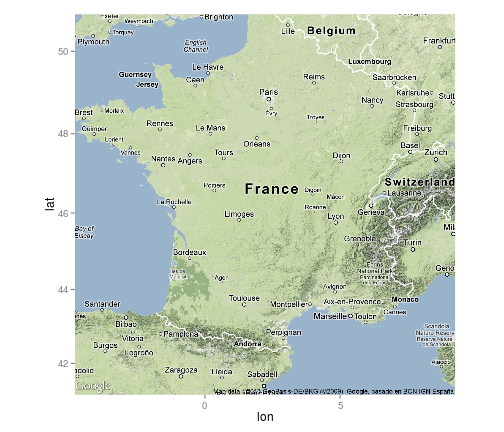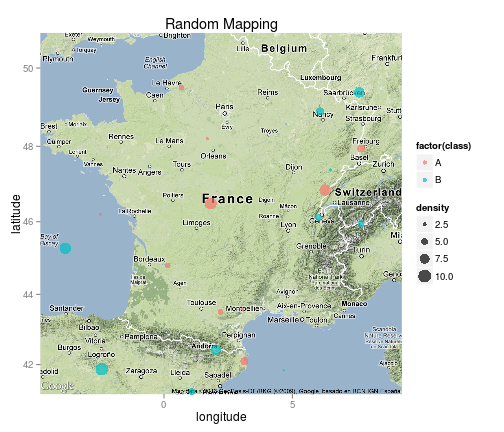Mapping in R using ggplot,

Making nice maps with great layout in R can be somewhat daunting, the library of ggplot allow us to draw nice and easy to customize plots. Well another library extend this ggplot to maps: ggmap. Here is a short overview of the utilities provided by ggmap:

library(ggmap)
# download a basic map of France, see ?get_map for the different map
# styles
fr <- get_map("France", zoom = 6, maptype = "terrain")
# plot
ggmap(fr)# create some random points with long/lat coordinates and some other
# variables
points <- data.frame(lon = rnorm(50, mean = 2.5, sd = 5), lat = rnorm(50, mean = 46,
sd = 8), class = sample(c("A", "B"), 50, replace = TRUE), density = sample(1:10,
50, replace = TRUE))
# and now we can do exactly what we did with ggplot
ggmap(fr) + geom_point(data = points, aes(lon = lon, lat = lat, color = factor(class),
size = density), alpha = 0.7) + labs(x = "longitude", y = "latitude", title = "Random Mapping")
## Warning: Removed 29 rows containing missing values (geom_point).This library interprete the map as colors on particular x/y coordinates a bit like the raster package do. R does not understand these as spatial objects, therefore care must be taken with the projection in which you are working with, the map from the get_map function are usually in long/lat coordinates. Some ressources: -A presentation done by the creator of the package: https://sites.google.com/site/davidkahle/ggmap

Funny mapping